# Class 10 Science: CBSE Sample Question Paper- Term I (2021-22) - 2 Notes | Study CBSE Sample Papers For Class 10 - Class 10

## Class 10: Class 10 Science: CBSE Sample Question Paper- Term I (2021-22) - 2 Notes | Study CBSE Sample Papers For Class 10 - Class 10

The document Class 10 Science: CBSE Sample Question Paper- Term I (2021-22) - 2 Notes | Study CBSE Sample Papers For Class 10 - Class 10 is a part of the Class 10 Course CBSE Sample Papers For Class 10.
All you need of Class 10 at this link: Class 10

Class-X
Time: 90 Minutes
M.M: 40

General Instructions:

1. The Question Paper contains three sections.
2. Section A has 24 questions. Attempt any 20 questions.
3. Section B has 24 questions. Attempt any 20 questions.
4. Section C has 12 questions. Attempt any 10 questions.
5. All questions carry equal marks.
6. There is no negative marking.
Section - A

Q.1: Choose the balanced equation (s) from the given.
(i) Ca(OH)2 + CO2 → CaCO3 + H2
(ii) Fe + CuSO4 → FeSO4 + Cu
(iii) KClO4 → KCl  + 2O
(iv) Cu +  2HNO3 → Cu(NO3)2 + 2NO2 + H2O
(a) I and II only
(b) I, II, and III only
(c) I, III and IV only
(d) All of these

Of the given options, only Equation IV is not balanced. The balanced equation is :

Cu + 4HNO3 → Cu(NO3)2 + 2NO2 + 2H2O

Q.2: In a chemical reaction, the substances that take part is called ____I_____ and the substance that is formed as a result of chemical reaction is called _____II_______.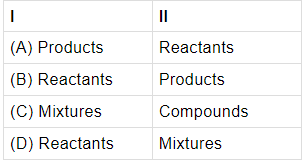(a) A
(b) B
(c) C
(d) D

Reactants are the substances that take part in a chemical reaction while products are formed during the reaction.

Q.3: The reaction in the given figure is an example of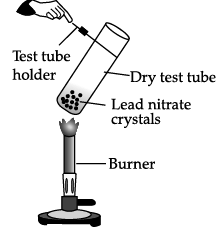(a) Combination Reaction
(b) Decomposition Reaction
(c) Double Decomposition Reaction
(d) Displacement Reaction

When lead nitrate is heated, it breaks down into lead monoxide, nitrogen dioxide and oxygen.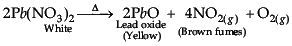The reaction is an example of decomposition reaction.

Q.4: Which of these equations is/are not balanced ?
(i) 2Al + 3H2SO4 → Al2(SO4)3 + 3H2
(ii) 2AlCl3 + 3Ca(OH)2 → 2Al(OH)3 + 3CaCl2
(iii) 2KClO3 → 2KCl + 3O2
(iv) Mg + H2O → Mg(OH)2 + H2
(a) II only
(b) IV only
(c) I and III only
(d) I, II and III only

The balanced equation is: Mg + 2H2O → Mg(OH)2 + H2

Q.5: Identify X in the given equation : MnO2 + 4HCl  MnCl2 + X + 2H2O
(a) Cl2
(b) O2
(c) Mn(OH)2
(d) 2Cl2

when manganese dioxide reacts with hydrochloric acid, the manganese dioxide (MnO2) reduces to MnCl2 while hydrochloric acid (HCl) oxidizes to chlorine gas (Cl2). So, X is Cl2. The chemical reaction is : MnO2  + 4HCl → MnCl+ Cl2 + 2H2O

Q.6: Sania found an element X, which reacts with oxygen and forms oxide of X which turns red litmus solution blue. The element X is a:
(a) Non-Metal
(b) Metal
(c) Mixture
(d) Alloy

All metals combine with oxygen to form metal oxides, that are basic in nature and  turns red litmus solution blue.

Q.7: Choose the incorrect statement?
(A) Aluminium when heated in air form aluminium oxide.
(B) Sodium metal reacts vigorously with acid to produce sodium chloride and hydrogen gas.
(C) Magnesium reacts with hot water to form magnesium hydroxide and hydrogen.
(D) Copper reacts with any form of water to form metal hydroxide.

Metals like lead, copper, silver and gold do not react with any form of water.

Q.8: Choose the incorrect statement about aqua-regia?
(a) It is a mixture of one part of concentrated nitric acid and three parts of concentrated hydrochloric acid.
(b) It is a mixture of one part of dilute nitric acid and three parts of concentrated sulphuric acid.
(c) It can dissolve all metals.
(d) Its meaning in Latin is royal water.

The meaning of Aqua regia in Latin is royal water. It is a freshly prepared mixture of concentrated hydrochloric acid and concentrated nitric acid in the ratio 3:1. It can dissolve gold metal which is least reactive metal.

Q.9: There are five metals given:   Zn, Sn, Ag, Na, Ca. Arrange the given metals from least reactive to most reactive.
(a) Sn<Na<Zn<Ca<Ag
(b) Ag<Zn<Sn<Na<Ca
(c) Ag<Sn<Zn<Ca<Na
(d) Zn<Sn<Ag<Ca<Na

The metals from least reactive to most reactive is: Ag<Sn<Zn<Ca<Na

Q.10: The compound which dissolves in water to give a solution with a pH greater than 7 is:
(a) Calcium Carbonate
(b) Copper (II) Hydroxide
(c) Sodium Hydroxide
(d) Sulphur Dioxide

Sodium hydroxide is an alkali that dissolves in water to form a solution of pH as greater than 7.

Q.11: Which cell organelles contain the light- absorbing green pigment?
(a) Mitochondria
(b) Ribosomes
(c) Chloroplast
(d) Nucleolus

Chloroplast in the leaf is the main site of photosynthesis. Chloroplast contains a green colour pigment called chlorophyll.

Q.12: Which one of the following is not present in the gastric juice secreted by stomach?
(a) Pepsin
(b) Renin
(c) Mucus
(d) Bile

Bile is dark green or a yellowish brown fluid which is produced by the liver and comes to the small intestine through hepato-pancreatic duct.

Q.13: Gas ‘P’ diffuses from a capillary into an alveolus in the lungs. The gas ‘P’ expelled out from the alveolus during: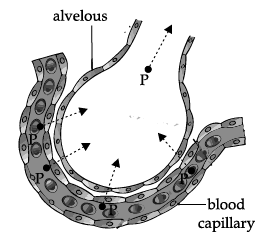(a) Inhalation
(b) Exhalation
(c) Respiration
(d) Inspiration

The gas that diffused out from a capillary into an alveolus is carbon dioxide. During exhalation carbon dioxide gas is expelled out.

Q.14: Based on the given information, X, Y and Z are : X- It transports blood to the heart.
Y- It connects the arteries and veins.
Z- It transports blood away from the heart.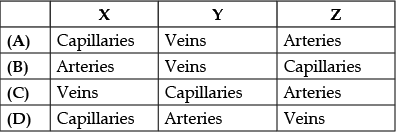(a) A
(b) B
(c) C
(d) D

In the given question X is vein that carry deoxygenated blood, Y is Capillaries and Z is arteries that carry oxygenated blood.

Q.15: Choose the correct statement about translocation.
(a) It is the continuous evaporation of water from the cells of a leaf.
(b) It transports food from the leaves.
(c) It binds carbon monoxide with haemoglobin.
(d) It diffuses carbon dioxide into the blood.

Translocation is the transport of soluble products of photosynthesis (food or glucose) from one part to the other parts of the plant.

Q.16: The correct sequence of the processes taking place in nutrition in animals is :
(a) Ingestion, Assimilation, Digestion, Absorption, Egestion
(b) Assimilation, Absorption, Ingestion, Digestion, Egestion
(c) Ingestion, Digestion, Absorption, Assimilation, Egestion
(d) Ingestion, Digestion, Assimilation, Absorption, Egestion

There are five steps in the process of nutrition in animals. These are: Ingestion, Digestion, Absorption, Assimilation and Egestion.

Q.17: Choose the incorrect statement.
(a) In primary rainbow, red colour is formed on the outside and violet colour on the inside.
(b) A normal human eye cannot clearly see all the objects at the different distances.
(c) A beam of white light gives a spectrum on passing through a hollow prism.
(d) Light rays of different colour travel with the same speed in vacuum and air.

A beam of white light gives a spectrum on passing through a glass prism. This phenomenon is called dispersion of light.

Q.18: If white light is incident on prism X then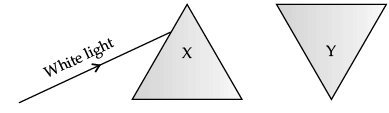(i) The ray of light which emerges out of the prism Y will be white light.
(ii) The refraction produced by the prism Y is equal and opposite to that produced by prism X.
Choose the correct statement (s).
(a) Only I
(b) Only II
(c) Both I and II
(d) None of these

When an inverted prism (Y) is placed in the path of dispersed light then after passing through the prism, they recombine to form white light.

Q.19: What will be the colour of an object, which can absorb all the seven colours but does not reflect even one?
(a) White
(b) Black
(c) Violet
(d) Red

When an object absorbs all the seven colours and reflects none, it looks black.

Q.20: The path of light beam becomes visible when a fine beam of sunlight enters a smoke filled room through a hole. It is because of:
(a) Reflection
(b) Refraction
(c) Atmospheric Refraction
(d) Scattering Effect

The smoke particles scatter the white light and hence the path of light beam becomes visible.

Q.21: If the earth had no atmosphere, the sky would appear ___________ in colour.
(a) Colourless
(b) White
(c) Blue
(d) Black

We can see the sky because the sunlight gets scattered by the particles present in the atmosphere. Without it, the sky would be dark and black with just the sun and the stars as bright objects in space.

Q.22: A red colour flower appears red because:
(a) It absorbs red colour.
(b) It allows red colour to pass through.
(c) It absorbs and refracts red colour.
(d) It reflects red colour.

A red coloured object appears to be red because it reflects red colour.

Q.23: Choose the correct option.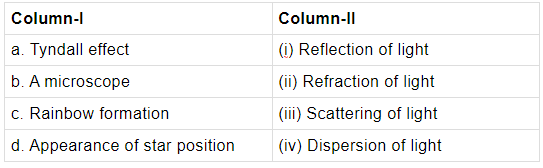(a) (iii) (i) (iv) (ii)
(b) (i) (iii) (ii) (iv)
(c) (ii) (iii) (iv) (i)
(d) (iv) (i) (iii) (ii)

Tyndall effect is due to scattering of light by the colloidal particles. A microscope uses a mirror to reflect light to the specimen under the microscope. Rainbow is formed due to dispersion of light and the appearance of star position than its actual position is due to atmospheric refraction.

Q.24: Given, the optical densities of four optical media P, Q, R and S.
P = 1.35
Q = 1.21
R = 1.58
S = 1.002
The light will travel fastest in _______I______ because its optical density is _______II________.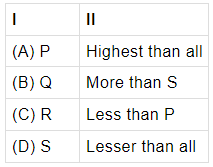(a) A
(b) B
(c) C
(d) D

The lesser the optical density, the more is the speed of light.

SECTION-B
Q.25: Identify “a” and “b” on the given equation.
aFe + bH2O → Fe2O4 + H2
(a) a is 3 and b is 4
(b) a is 4 and b is 3
(c) a is 2 and b is 5.
(d) a is 3 and b is 5

The balanced chemical equation is : 3Fe+ 4H2O → Fe3O4 + 4H2.

Q.26: Choose odd one out.
(a) Carbon + Oxygen → Carbon dioxide
(b) Hydrogen + Chlorine → Hydrogen chloride
(c) Glucose → Carbon dioxide + Water
(d) Sulphur + Oxygen → Sulphur dioxide

The digestion of food is a decomposition reaction, while the others are combination reactions.

Q.27: Which of the following are combination reactions?
(i)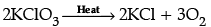(ii) MgO + H2O → Mg(OH)2
(iii) 4Al + 3O2 → 2Al2O3
(iv) Zn + FeSO4 → ZnSO4 + Fe.
(a) (i) and (iii)
(b) (iii) and (iv)
(c) (ii) and (iv)
(d) (ii) and (iii)

Combination reaction is a reaction in which two or more reactants react together to form a single product. In reaction
(ii) MgO and H2O combine to form Mg(OH)2 and in reaction
(iii), Al and Ocombine to form 2Al2O3. Reaction
(i) is decomposition reaction and reaction
(iv) is displacement reaction.

Q.28: During the preparation of hydrogen chloride gas on a humid day, the gas is usually passed through the guard tube containing calcium chloride. The role of calcium chloride taken in the guard tube is to
(a) Absorb the evolved gas.
(b) Moisten the gas.
(c) Absorb moisture from the gas.
(d) Absorb Cl ions from the evolved gas.

The role of calcium chloride taken in the guard tube is to absorb moisture from the gas. This is because calcium chloride is used as a drying agent which absorbs moisture from the hydrogen chloride (HCl) gas.

Q.29: Which of the following statements is not correct?
(a) All metal carbonates react with acid to give a salt, water and carbon dioxide
(b) All metal oxides react with water to give salt and acid
(c) Some metals react with acids to give salt and hydrogen
(d) Some non-metal oxides react with water to form an acid

Metal oxides are basic in nature. They form salts and water when react with acids except some amphoteric oxides like zinc oxide and aluminium oxide, thus, metal oxides are not salts. Therefore, all metal oxides react with water to give salt and acid is not correct.

Q.30: Which of the following statements is true for acids?
(a) Bitter and change red litmus to blue
(b) Sour and change red litmus to blue
(c) Sour and change blue litmus to red
(d) Bitter and change blue litmus to red

Property of acids is that it is sour in taste and change blue litmus to red.

Q.31: Assertion (A): When copper (II) oxide is heated with hydrogen, the copper metal and water are formed. This type of reaction is redox reaction.
CuO + H2 → Cu+ H2
Reason (R): In the given reaction H2 is oxidised to H2O and CuO is reduced to Cu.
(a) Both A and R are true and R is the correct explanation of A.
(b) Both A and R are true but R is NOT the correct explanation of A.
(c) A is true but R is false.
(d) A is false and R is true.

As oxidation and reduction are taking place simultaneously, it is called a redox reaction.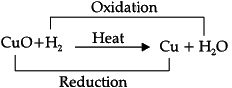Q.32: Assertion (A): Hydrogen is a metal and has been assigned a place in the reactivity series of metals.
Reason (R): It has tendency to lose electron and forms a positive  ion H+.
(a) Both A and R are true and R is the correct explanation of A.
(b) Both A and R are true but R is NOT the correct explanation of A.
(c) A is true but R is false.
(d) A is false and R is true.

Hydrogen is a metal which has been assigned a place in the reactivity series of metals because it has tendency to lose electrons and form a positive ion H+.

Q.33: Assertion (A): Valves are present in the arteries.
Reason (R): Arteries carry oxygenated blood from heart to different body parts except pulmonary artery.
(a) Both A and R are true and R is the correct explanation of A.
(b) Both A and R are true but R is NOT the correct explanation of A.
(c) A is true but R is false.
(d) A is false and R is true.

Valves are absent in arteries, whereas it is present in veins, which prevent back flow of blood.

Q.34: Assertion (A): Sun is visible 2 min before actual sunrise and 2 min after sunset.
Reason (R): It is because of atmospheric refraction.
(a) Both A and R are true and R is the correct explanation of A.
(b) Both A and R are true but R is NOT the correct explanation of A.
(c) A is true but R is false.
(d) A is false and R is true.

The sun appears about two minutes earlier than actual sunrise and the sun remains visible for about two minutes after actual sunset. It is because of refraction. When the sun is below the horizon, the rays have to pass from rarer to denser medium. So, rays bend towards the normal. As a result the sun appears higher than its actual position.

Q.35: Aluminium is used for making cooking utensils. Which of the following properties of aluminium are responsible for the same?
(i) Good thermal conductivity
(ii) Good electrical conductivity
(iii) Ductility
(iv) High melting point

(a) (i) and (ii)
(b) (i) and (iii)
(c) (ii) and (iii)
(d) (i) and (iv)

Metals are good conductors of heat and have high melting points. Thus, Aluminium is used for making utensils.

Q.36: Which of the following oxide(s) of iron would be obtained on prolonged reaction of iron with steam?
(A) FeO
(B) Fe2O3
(C) Fe3O4
(D) Fe2O3 and Fe3O4

Iron reacts only with steam to form a metal oxide and hydrogen.
3 Fe(s) + 4 H2O(g) → Fe3O4(s) + 4 H2(g)

Q.37: The given experimental set up shows that :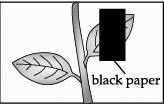(a) Respiration takes place in leaves.
(b) Transpiration takes place in leaves

(c) Sunlight is essential for the process of photosynthesis.
(d) Trophic movements take place in leaves.

The aim of the given experiment is used to show that sunlight is essential for photosynthesis.

Q.38: Which of the parts of the circulatory system has the highest concentration of oxygen?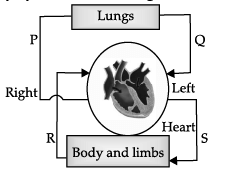(a) P and Q
(b) Q and S
(c) R and P
(d) S and R

Q and S have the highest concentration of oxygen in the blood.

Q.39: _____ is the structural and functional unit of the excretory system.
(a) Neuron
(b) Nephron
(c) Alveolus
(d) Cell

Nephron is the structural and functional unit of excretory system.

Q.40: Study the given statements.
(i) The component “P” transports digested food.
(ii) The component “Q” helps in clotting of blood.
Identify P and Q.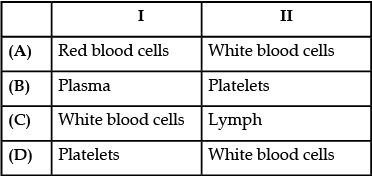(a) A
(b) B
(c) C
(d) D

Plasma transports digested food to the cells and platelets help in blood clotting.

Q.41: _____ is the common phase between aerobic and anaerobic respiration.
(a) Glycolysis
(b) Krebs's cycle
(c) TCA cycle
(d) EMP pathway

Glycolysis is the common phase between aerobic and anaerobic respiration.

Q.42: A mirror has a focal length of + 15 cm. It is a :
(a) Convex mirror
(b) Concave mirror
(c) Plane mirror
(d) None of these

It is a  convex mirror. The pole, focus, focal length  and  centre of curvature, all are behind the mirror and as we know that when it is behind the mirror it will be positive.

Q.43: When a convergent beam of light is incident on a plane mirror, then image formed is _____ and _____.
(a) Upright and real
(b) Upright and virtual
(c) Inverted and virtual
(d) Inverted and real

When a convergent beam of light is incident on a plane mirror, then image formed is inverted and virtual.

Q.44: Which of these quantities remains constant during the refraction of light ?
(a) Wavelength
(b) Speed
(c) Frequency
(d) Velocity

During the refraction of light wavelength and speed change. Frequency is always a constant quantity.

Q.45: In case of concave mirror, the distance between a real object and its real image, when the object is placed at the centre of curvature is :
(A) F
(B) 2F
(C) 4F
(D) Zero

When the object is placed at C of a concave mirror, the distance between a real object and its real image is zero.

Q.46: _____ always produces a diminished image of an object.
(a) A convex mirror
(b) A concave mirror
(c) A convex lens
(d) Bi-convex lens

A convex mirror always produces only virtual and diminished images of objects.

Q.47: The correct lens formula is :
(A) 1/f = 1/u − 1/v
(B) 1/f = 1/u + 1/v
(C) 1/f = 1/v − 1/u
(D) 1/v = 1/u − 1/f

The correct lens formula is :
1/v - 1/u = 1/f

Q.48: The term used to refer the extent to which image of an object can be magnified is :
(A) Refraction
(B) Refractive Index
(C) Magnification
(D) Dispersion

The term used to refer the extent to which image of an object can be magnified is magnification.

SECTION-C
Q.49: Study the given diagram and answer any of the following questions.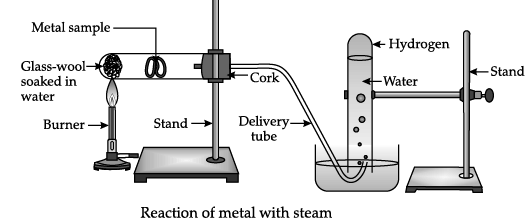The metal sample in the above diagram can be:
(a) Aluminium
(c) Copper
(d) Silver

Metals like iron, zinc and aluminium do not react with cold or hot water. They react with steam to form corresponding hydroxide and hydrogen gas. Metals like lead, copper, silver and gold do not react with any form of water.

Q.50: Study the given diagram and answer any of the following questions.Which of these gases is released when metals react with water?
(a) Nitrogen
(b) Oxygen
(c) Hydrogen
(d) Chlorine

Metals react with water to produce metal oxide and hydrogen gas.

Metal oxides that are soluble in water dissolve in water to form metal hydroxide.

Metal + Water → Metal oxide + Hydrogen Metal oxide + Water → Metal hydroxide

Q.51: Study the given diagram and answer any of the following questions.Which of the following is the test for the above gas released?
(a) Relights a glowing splint
(b) Turns limewater ‘milky’ (white precipitate)
(c) Turns blue litmus red and then leaches it
(d) Gives a squeaky pop with a lit splint

Characteristic test for hydrogen (H2) gas can be performed by bringing a burning candle near the source of hydrogen. On doing so, hydrogen gas burns with a squeaky pop sound. Hydrogen gas is recognized by the ‘pop’ when it burns. The ‘pop’ is the sound of a small explosion.

Q.52: Study the given diagram and answer any of the following questions.The reactivity order of metals towards water is:
(a) Zn > Fe > Al > Na > Ca
(b) Na > Ca > Zn > Fe > Al
(c) Al > Na > Ca > Zn > Fe
(d) Fe > Al > Na > Ca > Zn

The order of reactivity with water in descending order  is Na > K > Ca > Zn > Fe > Al  Sodium and potassium react violently with the release of huge amount of heat. So the reaction is highly exothermic. Hydrogen gas which is produced during the reaction catches fire.  Calcium reacts less violently with water. The heat evolved is not sufficient for the hydrogen to catch fire. Instead, calcium floats over water because bubbles of hydrogen gas stick to the metal surface Aluminium, iron and zinc react with steam to form respective oxide and hydrogen gas. Thus, metals react with water to form metal hydroxide and hydrogen gas, which we can check by placing a match-stick near it.

Q.53: The given diagram shows a model of a nephron and its associated blood vessels. Study the diagram and answer the following questions.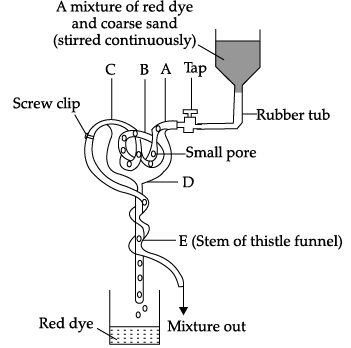Which one of the following letters represents glomerulus in the model?
(a) D
(b) E
(c) B
(d) C

The label A is Renal artery, B is Glomerulus, C is Renal tubule and D is Bowman’s capsule.

Q.54: The given diagram shows a model of a nephron and its associated blood vessels. Study the diagram and answer the following questions.Each nephron has a cup shaped upper end called ________I______ , which is labeled as _____II____ in the model.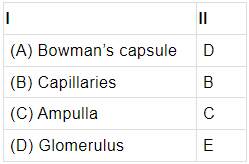(a) A
(b) B
(c) C
(d) D

The upper cup shaped end of a nephron is called Bowman’s capsule. It contains glomerulus which is a group of blood capillaries.

Q.55: The given diagram shows a model of a nephron and its associated blood vessels. Study the diagram and answer the following questions.This model has been criticized for being a poor representation of a nephron. Which of these statements explain(s) this?
(i) The rubber tubing at B is not split up into numerous branches like the capillary network of the glomerulus.
(ii) The rubber tubing at B should be continuous and not contain small pores.
(iii) The stem of the thistle funnel (E) is not coiled to represent the convoluted tubules of the nephron.

(a) I only
(b) I and III only
(c) II and III only
(d) I, II and III

The rubber tubing at B is not split up into numerous branches like the capillary network of the glomerulus. Also, the stem of the thistle funnel (E) is not coiled to represent the convoluted tubules of the nephron.

Q.56: The given diagram shows a model of a nephron and its associated blood vessels. Study the diagram and answer the following questions.The diagram shows a kidney tubule and some of its associated blood vessels.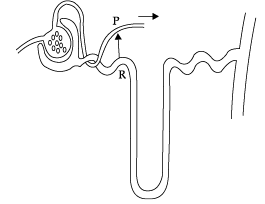Which substance is entirely reabsorbed from the fluid at R to the blood at P?

(a Glucose
(b) Salts
(c) Urea
(d) Water

In the diagram R represents proximal convoluted tubule. The proximal convoluted tubule avidly reabsorbs filtered glucose into the peritubular capillaries so that it is all reabsorbed by the end of the proximal tubule.

Q.57: When lights of all colours are mixed in a certain proportion, the result is a colour less light, called white light. Sunlight is an example of white light. It is a mixture of lights of seven main colours. On refraction, lights of different colours bend by different amounts. So, when light made up of different colours gets refracted, its components tend to separate, or split. Normally, after refraction, the separation between the component colours of light is so little that they are not visible. But if the components get bent very sharply, the separated colours become visible. For example, when white light falls at certain angles on a glass prism, it splits into its seven component colours marked 1 to 7 as shown in figure. The various colours seen are Violet, Indigo, Blue, Green, Yellow, Orange and Red. The sequence of colour can be remembered as VIBGYOR.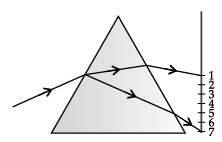Based on the above diagram, which of the following statements is correct?
(a) The colour at position marked 5 is similar to the colour of gold metal.
(b) The colour at position marked 2 has longer wavelength than 1.
(c) The colour at position marked 7 is violet.
(d) The colour at position marked 5 has higher speed than 1.

The colour at position marked 7 is violet. Red is the least deviated colour as it has largest/ longest wavelength and violet is the most deviated colour as it has smallest wavelength in visible spectrum.Q.58: When lights of all colours are mixed in a certain proportion, the result is a colour less light, called white light. Sunlight is an example of white light. It is a mixture of lights of seven main colours. On refraction, lights of different colours bend by different amounts. So, when light made up of different colours gets refracted, its components tend to separate, or split. Normally, after refraction, the separation between the component colours of light is so little that they are not visible. But if the components get bent very sharply, the separated colours become visible. For example, when white light falls at certain angles on a glass prism, it splits into its seven component colours marked 1 to 7 as shown in figure. The various colours seen are Violet, Indigo, Blue, Green, Yellow, Orange and Red. The sequence of colour can be remembered as VIBGYOR.Choose the correct statement.
(a) The colours in the order of increasing wavelength are 1 < 2 < 3 < 4 < 5 < 6 < 7.
(b) The colours in the order of increasing frequency are 1 < 2 < 3 < 4 < 5 < 6 < 7.
(c) The colours in the order of decreasing speed are 7 > 6 > 5 > 4 > 3 > 2 > 1.
(d) The colours in the order of decreasing deviation are 1 > 2 > 3 > 4 > 5 > 6 > 7.

Red, orange, yellow, green, blue, indigo and violet are the seven colours of the white light spectrum in order of their increasing frequencies.

Q.59: When lights of all colours are mixed in a certain proportion, the result is a colour less light, called white light. Sunlight is an example of white light. It is a mixture of lights of seven main colours. On refraction, lights of different colours bend by different amounts. So, when light made up of different colours gets refracted, its components tend to separate, or split. Normally, after refraction, the separation between the component colours of light is so little that they are not visible. But if the components get bent very sharply, the separated colours become visible. For example, when white light falls at certain angles on a glass prism, it splits into its seven component colours marked 1 to 7 as shown in figure. The various colours seen are Violet, Indigo, Blue, Green, Yellow, Orange and Red. The sequence of colour can be remembered as VIBGYOR.When a white light falls on a prism, the ray at its first surface suffers:
(a) No Refraction
(b) Dispersion Only
(c) Deviation Only
(d) Both (B) and (C)

When a white light ray falls on the first surface of a prism, light rays of different colours due to their different speeds in glass get refracted (or deviated) through different angles. Thus, the dispersion of white light into its constituent colours takes place at the first surface of prism.

Q.60: When lights of all colours are mixed in a certain proportion, the result is a colour less light, called white light. Sunlight is an example of white light. It is a mixture of lights of seven main colours. On refraction, lights of different colours bend by different amounts. So, when light made up of different colours gets refracted, its components tend to separate, or split. Normally, after refraction, the separation between the component colours of light is so little that they are not visible. But if the components get bent very sharply, the separated colours become visible. For example, when white light falls at certain angles on a glass prism, it splits into its seven component colours marked 1 to 7 as shown in figure. The various colours seen are Violet, Indigo, Blue, Green, Yellow, Orange and Red. The sequence of colour can be remembered as VIBGYOR.What is the wavelength range of white light?
(a) 4 nm to 8 nm
(b) 40 nm to 80 nm
(c) 400 nm to 800 nm
(d) 4000 nm to 8000 nm

The wavelength range of white light is 400 nm to 800 nm.

The document Class 10 Science: CBSE Sample Question Paper- Term I (2021-22) - 2 Notes | Study CBSE Sample Papers For Class 10 - Class 10 is a part of the Class 10 Course CBSE Sample Papers For Class 10.
All you need of Class 10 at this link: Class 10Use Code STAYHOME200 and get INR 200 additional OFF

## CBSE Sample Papers For Class 10

174 docs|7 tests

Track your progress, build streaks, highlight & save important lessons and more!

,

,

,

,

,

,

,

,

,

,

,

,

,

,

,

,

,

,

,

,

,

;